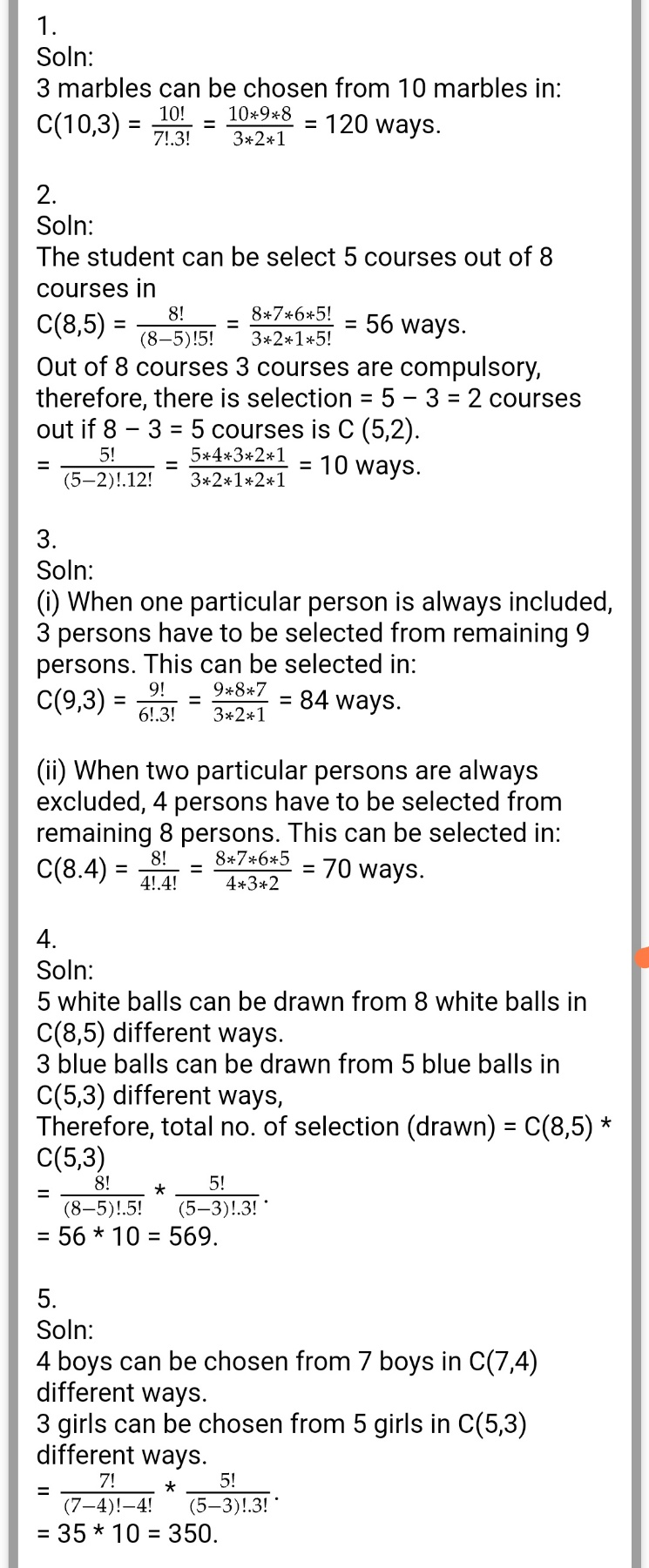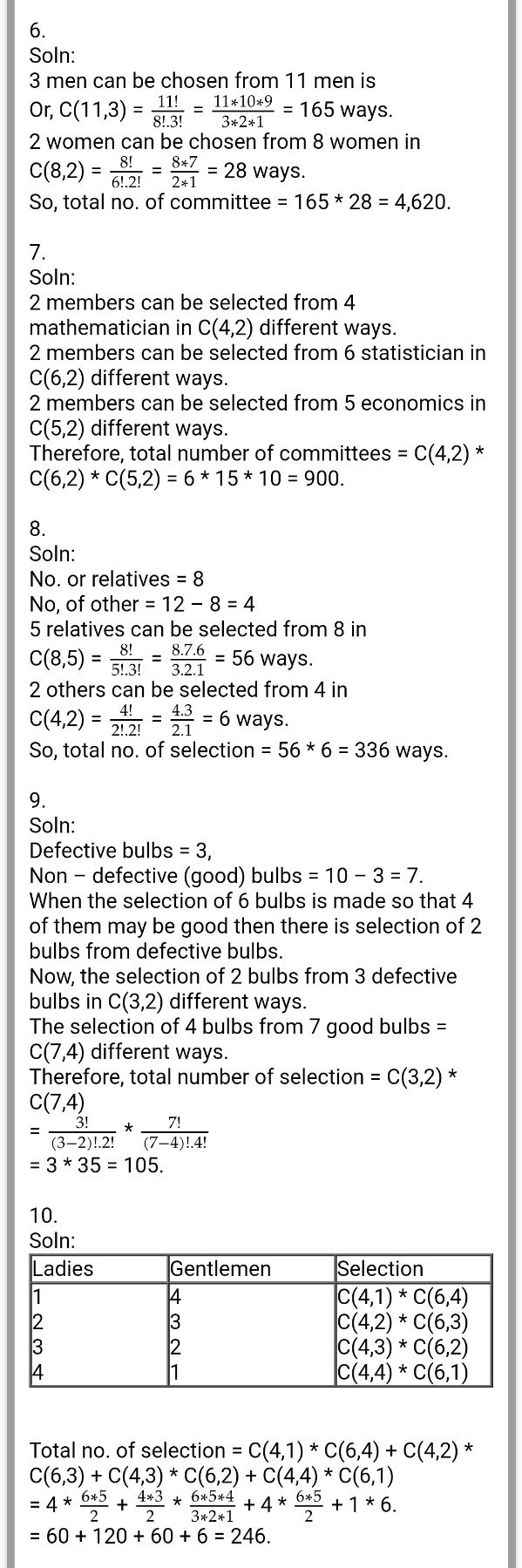# Permutation & Combination - Exercise 1.3 : Class 12 Math

Permutation and Combination - Exercise 1.3 : Class 12 Mathematics. Complete Exercise 1.3 of Class 12 Math, NEB 2080.

## Chapter 1: Permutation and Combination.

### Exercise: 1.3

Complete Exercise of Permutation and Combination - Exercise 1.3 : Class 12 Mathematics 2080 NEB.

Read: NEB Class 12 Mathematics All Chapter ExercisePermutation & Combination - Exercise 1.1 : Class 12 Math

Permutation & Combination - Exercise 1.2 : Class 12 Math

Getting Info...